# Force

In physics, force is defined as the rate of change of momentum of an object. This definition was given by Isaac Newton in the seventeenth century. In simpler terms, force may be thought of as an influence that may cause an object to accelerate. Force and mass are fundamental to Newtonian physics.

In everyday life, a force may be experienced in various ways, such as a lift, a push, or a pull. A familiar example of force is the weight of an object, which is defined as the amount of gravitational force exerted on the object. In addition, a force (or combination of forces) may cause an object to rotate or become deformed. Rotational effects and deformation are determined respectively by the torques and stresses that the forces create.

In the twentieth century, it was found that all known forces could be reduced to four fundamental forces: the strong force, weak force, electromagnetic force, and gravity. However, contemporary physics such as quantum mechanics and general relativity no longer regard the concept of force as fundamental. In quantum mechanics, force is seen as derivative of the interactions between particles. In general relativity, gravitational force is a trajectory along curved space-time.

## History

Aristotle and his followers believed that it was the natural state of objects on Earth to be motionless and that they tended toward that state if left alone. But this theory, although based on the everyday experience of how objects move, was first shown to be unsatisfactory by Galileo as a result of his work on gravity. Galileo constructed an experiment in which stones and cannonballs were both rolled down an incline to disprove the Aristotelian theory of motion early in the seventeenth century. He showed that the bodies were accelerated by gravity to an extent that was independent of their mass and argued that objects retain their velocity unless acted on by a force—usually friction.

Isaac Newton is recognized as having given the mathematical definition of force as the rate of change (time derivative) of momentum. In 1784, Charles Coulomb discovered the inverse square law of interaction between electric charges using a torsion balance.

With the development of quantum field theory and general relativity in the twentieth century, it was realized that particles influence one another through fundamental interactions, and that "force" is a concept arising from the conservation of momentum. Only four fundamental interactions are known. They are called the strong, electromagnetic, weak, and gravitational interactions (in order of decreasing strength). In the 1970s, the electromagnetic and weak interactions were unified into the "electroweak interaction."

## Definition

Force is defined as the rate of change of momentum with time: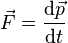$\vec{F} = {\mathrm{d}\vec{p} \over \mathrm{d}t}$

The quantity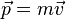$\vec{p} = m \vec{v}$ (where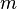$m\,$ is the mass and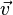$\vec{v}$ is the velocity) is called the momentum. This is the only definition of force known in physics.

Momentum is a vector quantity—that is, it has both a magnitude and direction. Therefore force is also a vector quantity. The actual acceleration of the body is determined by the vector sum of all forces acting on it (known as net force or resultant force).

If the mass m is constant in time, then Newton's second law can be derived from this definition: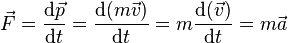$\vec{F} = \frac{\mathrm{d}\vec{p}}{\mathrm{d}t}= \frac{\mathrm{d}(m\vec{v})}{\mathrm{d}t} = m\frac{\mathrm{d}(\vec{v})}{\mathrm{d}t} = m\vec{a}$

where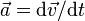$\vec{a} = {\mathrm{d} \vec{v}} /{\mathrm{d}t}$ (the rate of change of velocity) is the acceleration.

This is the form Newton's second law is usually taught in introductory physics courses.

All known forces of nature are defined via the above Newtonian definition of force. For example, weight (force of gravity) is defined as mass times acceleration of free fall: w = mg

It is not always the case that m, the mass of an object, is independent of time, t. For example, the mass of a rocket decreases as its fuel is burned. Under such circumstances, the above equation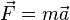$\vec{F} = m\vec{a}$ is obviously incorrect, and the original definition of force:$\vec{F} = {\mathrm{d}\vec{p} \over \mathrm{d}t}$ must be used.

Because momentum is a vector, then force is also a vector—it has magnitude and direction. Vectors (and thus forces) are added together by their components. When two forces act on an object, the resulting force, often called the resultant, is the vector sum of the original forces. This is called the principle of superposition. The magnitude of the resultant varies from the difference of the magnitudes of the two forces to their sum, depending on the angle between their lines of action. As with all vector addition, this results in a parallelogram rule: the addition of two vectors represented by sides of a parallelogram gives an equivalent resultant vector, which is equal in magnitude and direction to the transversal of the parallelogram. If the two forces are equal in magnitude but opposite in direction, then the resultant is zero. This condition is called static equilibrium, with the result that the object remains at its constant velocity (which could be zero).

As well as being added, forces can also be broken down (or "resolved"). For example, a horizontal force pointing northeast can be split into two forces, one pointing north, and one pointing east. Summing these component forces using vector addition yields the original force. Force vectors can also be three-dimensional, with the third (vertical) component at right-angles to the two horizontal components.

## Examples

• An object is in free fall. Its momentum changes as dp/dt = mdv/dt = ma = mg (if the mass, m, is constant), thus we call the quantity mg a "gravitational force" acting on the object. This is the definition of weight (w=mg) of an object.
• An object on a table is pulled downward toward the floor by the force of gravity. At the same time, the table resists the downward force with equal upward force (called the normal force), resulting in zero net force, and no acceleration. (If the object is a person, he actually feels the normal force acting on him from below.)
• An object on a table is gently pushed in a sideways direction by a finger. However, it doesn't move because the force of the finger on the object is now opposed by a force of static friction, generated between the object and the table surface. This force exactly balances the force exerted on the object by the finger, and no acceleration occurs. The static friction increases or decreases automatically. If the force of the finger is increased (up to a point), the opposing sideways force of static friction increases exactly to the point of perfect opposition.
• An object on a table is pushed by a finger hard enough that static friction cannot generate sufficient force to match the force exerted by the finger, and the object starts sliding across the surface. If the finger is moved with a constant velocity, it needs to apply a force that exactly cancels the force of kinetic friction from the surface of the table and then the object moves with the same constant velocity. Here it seems to the naive observer that application of a force produces a velocity (rather than an acceleration). However, the velocity is constant only because the force of the finger and the kinetic friction cancel each other. Without friction, the object would continually accelerate in response to a constant force.
• An object reaches the edge of the table and falls. Now the object, subjected to the constant force of its weight, but freed of the normal force and friction forces from the table, gains in velocity in direct proportion to the time of fall, and thus (before it reaches velocities where air resistance forces becomes significant compared to gravity forces) its rate of gain in momentum and velocity is constant. These facts were first discovered by Galileo.

## Types of Force

Although there are apparently many types of forces in the universe, they are all based on four fundamental forces, mentioned above. The strong and weak forces only act at very short distances and are responsible for holding certain nucleons and compound nuclei together. The electromagnetic force acts between electric charges, and the gravitational force acts between masses.

All other forces are based on these four. For example, friction is a manifestation of the electromagnetic force (acting between the atoms of two surfaces) and the Pauli exclusion principle, which does not allow atoms to pass through each other. The forces in springs modeled by Hooke's law are also the result of electromagnetic forces and the exclusion principle acting together to return the object to its equilibrium position. Centrifugal forces are acceleration forces (inertia forces) that arise simply from the acceleration of rotating frames of reference.

The modern quantum mechanical view of the first three fundamental forces (all except gravity) is that particles of matter (fermions) do not directly interact with each other but rather by the exchange of virtual particles (bosons). This exchange results in what we call electromagnetic interactions. (Coulomb force is one example of electromagnetic interaction).

In general relativity, gravitation is not strictly viewed as a force. Rather, objects moving freely in gravitational fields simply undergo inertial motion along a straight line in curved space-time — defined as the shortest space-time path between two points. This straight line in space-time is a curved line in space, and it is called the ballistic trajectory of the object. For example, a basketball thrown from the ground moves in a parabola shape, as it is in a uniform gravitational field. Similarly, planets move in ellipses, as they are in an inverse square gravitational field. The time derivative of the changing momentum of the body is what we label as "gravitational force."

### Force in Special Relativity

In the special theory of relativity, mass and energy are equivalent (as can be seen by calculating the work required to accelerate a body). When an object's velocity increases, so does its energy and hence its mass equivalent (inertia). It thus requires a greater force to accelerate it the same amount than it did at a lower velocity. The definition$\vec{F} = \mathrm{d}\vec{p}/\mathrm{d}t$ remains valid, but the momentum must be redefined (in order to be conserved) as:$\vec{p} = \frac{m\vec{v}}{\sqrt{1 - v^2/c^2}}$

where$v$ is the velocity and$c$ is the speed of light.

Note that this definition is consistent with the classic definition of momentum (mv) at low speeds.

Also, according to the theory of relativity, for objects moving at extremely high speeds, a constant force produces not a constant acceleration but an ever-decreasing acceleration as the object approaches the speed of light.

## Units of Measurement

The SI unit used to measure force is the newton (symbol N) where: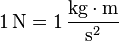$1\, \mathrm{N}=1\, \frac{\mathrm{kg} \cdot \mathrm{m}}{\mathrm{s}^{2}}$.

A newton is the amount of force required to accelerate a body with a mass of one kilogram at a rate of one meter per second squared.

A pound-force (lbf or lbf) is another common unit of force. One pound-force is the force equivalent to that exerted on a mass of one pound on the surface of Earth. When the standard g (an acceleration of 9.80665 m/s²) is used to define pounds force, the mass in pounds is numerically equal to the weight in pounds force. However, even at sea level on Earth, the actual acceleration of free fall is variable, over 0.53% more at the poles than at the equator.

The kilogram-force is a unit of force that was used in various fields of science and technology. In 1901, the CGPM improved the definition of the kilogram-force, adopting a standard acceleration of gravity for the purpose, and making the kilogram-force equal to the force exerted by a mass of 1 kg when accelerated by 9.80665 m/s². The kilogram-force is not a part of the modern SI system, but is still used in applications such as:

• Thrust of jet and rocket engines
• Spoke tension of bicycles
• Draw weight of bows
• Torque wrenches in units such as "meter kilograms" or "kilogram centimeters" (the kilograms are rarely identified as units of force)
• Engine torque output (kgf·m expressed in various word orders, spellings, and symbols)
• Pressure gauges in "kg/cm²" or "kgf/cm²"

Another unit of force called the poundal (pdl) is defined as the force that accelerates 1 lbm at 1 foot per second squared. Given that 1 lbf = 32.174 lb times one foot per second squared, we have 1 lbf = 32.174 pdl.

### Conversion Factors

Below are several conversion factors for measuring force in various units:

• 1 kgf (kilopond kp) = 9.80665 newtons
• 1 lbf = 4.448222 newtons
• 1 lbf = 32.174 poundals
• 1 kgf = 2.2046 lbf
• 1 dyne = 10-5 newtons
• 1 slug = 32.174 lb